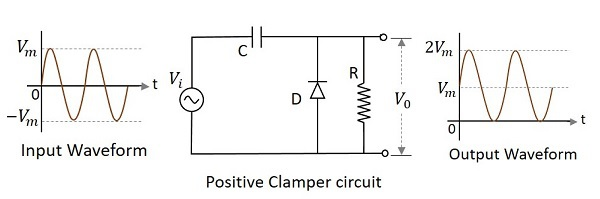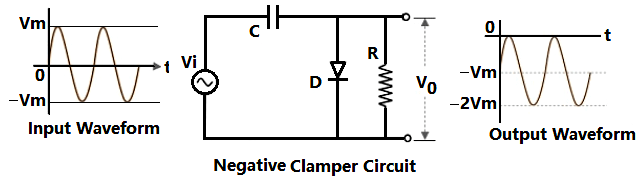### Clamper Circuit

The circuit which does not change the shape of waveform but they shift the wave form by adding DC value There are two types of clamper circuit.

1. Positive clapper.

2. Negative clamper.

1. Positive Clapper:1. Negative Clapper:Important Point:

1.  In ideal clamper circuit capacitor charges  through through diode where  as there is no   discharge  path to the  capacitor Hence the as input voltage changes the capacitor voltage does not changes  hence we do not get perfect champing circuit.

2. In practical clamper a resistance is added in parallel to the diode to provide discharging path to the capacitor the value of resistance should be large so that discharge through is very slow.

3. The discharge time constant RC such that RC >> T where T is the time period of Vin.